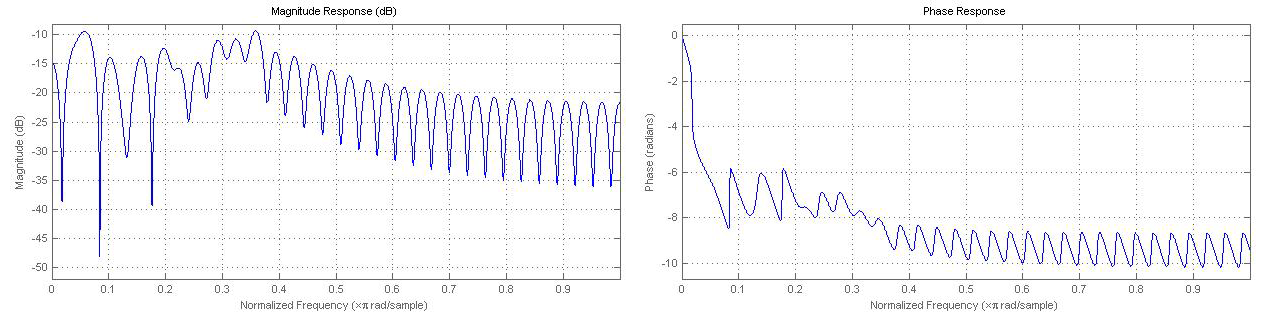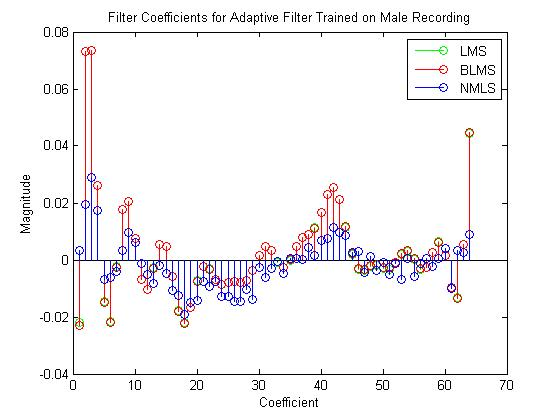# 0.3 The adaptive filter method

 Page 1 / 1
Background on a few adaptive filtering algorithms we tried in our echo cancellation project.

Adaptive filters are time-varying filters that use an update algorithm to create a finite impulse response (FIR) filter that outputs the best approximation of desired signal. Adaptive filters take in a desired signal and an input signal and update their parameters using feedback driven by the error signal. The adaptive filter finds the ideal filter coefficients for a filter that gives the least mean square of the error signal. The error signal is defined as the difference of the desired signal and the output signal.

$e\left(n\right)=d\left(n\right)-y\left(n\right)$

At every iteration (or block of samples) the gradient of the mean square error at that time is found, and is then used to adjust the filter coefficients at the time to converge towards the ideal filter coefficients.

We trained adaptive filters using signals recorded in a low-echo environment using three algorithms to filter out echoes in an echoic room and in the OEDK classroom. Our desired signal is a recording of our signal in a low-echo environment that would give us a signal with minimal echo. This gave us a baseline for the distortion of the signal caused by our equipment.

Having gathered a baseline signal, we recorded our input signals in the echoic room and the OEDK classroom. The basic idea of the adaptive filter method is to take in a desired signal and an input signal and mimic an ideal filter with ideal filter coefficients that would make the output signal virtually echo-free and therefore comparable to the desired signal. We explored three different adaptive filtering algorithms for our project.

## The least mean squares (lms) algorithm

The LMS algorithm is a class of adaptive filter used to mimic a desired filter by finding the filter coefficients that relate to producing the least mean squares of the error signal (the difference between the desired and the actual signal). The algorithm begins by assuming small weights (zero in most cases), and at each step, by finding the gradient of the mean square error, $E\left[{e}^{2}\left(n\right)\right]$ , the weights are updated using this cost function. Each iteration uses three steps:

• Calculate the output of the filter.
$y\left(n\right)={w}^{T}\left(n\right)x\left(n\right)$
• Calculate the estimate of the error using the error signal.
$e\left(n\right)=d\left(n\right)-y\left(n\right)$
• Update the weights of the FIR coefficients.
$w\left(n+1\right)=w\left(n\right)+2\mu e\left(n\right)x\left(n\right)$
$y\left(n\right)$ is the output of the filter, $w\left(n\right)$ are the coefficients of the FIR filter, $x\left(n\right)$ is the input signal, $d\left(n\right)$ is the desired signal, and $\mu$ is the step size.

Computational complexity: $2N$ additions, $2N+1$ multiplications.

The MathWorks adaptfilt.lms documentation can be used in Matlab to create an LMS adaptive filter object.

## The normalized lms (nlms) algorithm

NLMS allows for a variable step size $\mu \left(n\right)$ for each iteration. The first two steps of the algorithm are identical to the first two steps of the regular LMS algorithm.

• Calculate the output of the filter.
$y\left(n\right)={w}^{T}\left(n\right)x\left(n\right)$
• Calculate the estimate of the error using the error signal.
$e\left(n\right)=d\left(n\right)-y\left(n\right)$
• Calculate the step size for the input.
$\mu \left(n\right)=\frac{1}{{x}^{T}\left(n\right)x\left(n\right)}$
• Update the weights of the FIR coefficients.
$w\left(n+1\right)=w\left(n\right)+\mu \left(n\right)e\left(n\right)x\left(n\right)$

Computational Complexity: $2N$ additions $3N+1$ multiplications.

The MathWorks adaptfilt.nlms documentation can be used in Matlab to create an NLMS adaptive filter object.

## The block lms (blms) algorithm

Rather than computing by discrete samples, the BLMS algorithm calculates in blocks of data, reducing computation cost and improving the convergence rate. The BLMS algorithm can be implemented in the frequency domain using the Discrete Fourier Transform or the FFT.

Computational complexity: $\frac{4N}{B}+\left(\frac{N}{B}+3\right)\mathrm{log}\left(2B\right)$ , where $B$ is the block length.

The MathWorks adaptfilt.blms documentation can be used in Matlab to create a BLMS adaptive filter object.This particular filter is a BLMS trained on the male voice signal. Notice that the unit circle is in the region of convergence in the pole/zero plot (the filter is BIBO stable).

## Filtering in matlab

1. Choose a signal to be recorded. We chose a pseudo-dirac, an audiobook recording from The Hunger Games and a recording from Winston Churchill's A History of the English Peoples .
2. Record the signal being played in a room with desired acoustical properties. This is the desired signal, $d\left(n\right)$ . We chose a carpeted room in Hanszen College that had little discernible echo.
3. Record the signal being played in the echoed room. This is the input signal $x\left(n\right)$ . We recorded in the OEDK classroom and in an even more echoic classroom in Wiess College.
4. Import both responses into Matlab.
5. If you use Matlab's built-in adaptive filters. Create the adaptfilt object corresponding to the algorithm with desired parameters.
6. Use the maxstep() function to check that your filter is stable. If not, adjust your step size.
7. Use the filter() with your filter object, the input signal and the desire signal.
The desired signal, $d\left(n\right)$ , needs to be recorded in another room in order to neutralize the effects of the recording equipment and the amplifier. Using the original signal could lead to the filter trying to compensate for effects introduced by the equipment and the amplifier instead of the echoes caused by the room. Furthermore, the desired and input signals need to start at the same time. Cut out any silence beforehand so that the vectors line up, this way the adaptive filter sifts out the echoes at the appropriate times.
We used the Audacity software to record the sounds, trim the beginning of the sound files, and zero pad them to equal length. We exported these files as .wav files and then, on Matlab, used the wavread() function to import the sounds.
Links to MathWorks documentation for various adaptive filters can be found here .With the same training set (desired signal) each algorithm yields different filter coefficients. Step size: .0006. BLMS block length: 64.

where we get a research paper on Nano chemistry....?
what are the products of Nano chemistry?
There are lots of products of nano chemistry... Like nano coatings.....carbon fiber.. And lots of others..
learn
Even nanotechnology is pretty much all about chemistry... Its the chemistry on quantum or atomic level
learn
da
no nanotechnology is also a part of physics and maths it requires angle formulas and some pressure regarding concepts
Bhagvanji
Preparation and Applications of Nanomaterial for Drug Delivery
revolt
da
Application of nanotechnology in medicine
what is variations in raman spectra for nanomaterials
I only see partial conversation and what's the question here!
what about nanotechnology for water purification
please someone correct me if I'm wrong but I think one can use nanoparticles, specially silver nanoparticles for water treatment.
Damian
yes that's correct
Professor
I think
Professor
Nasa has use it in the 60's, copper as water purification in the moon travel.
Alexandre
nanocopper obvius
Alexandre
what is the stm
is there industrial application of fullrenes. What is the method to prepare fullrene on large scale.?
Rafiq
industrial application...? mmm I think on the medical side as drug carrier, but you should go deeper on your research, I may be wrong
Damian
How we are making nano material?
what is a peer
What is meant by 'nano scale'?
What is STMs full form?
LITNING
scanning tunneling microscope
Sahil
how nano science is used for hydrophobicity
Santosh
Do u think that Graphene and Fullrene fiber can be used to make Air Plane body structure the lightest and strongest. Rafiq
Rafiq
what is differents between GO and RGO?
Mahi
what is simplest way to understand the applications of nano robots used to detect the cancer affected cell of human body.? How this robot is carried to required site of body cell.? what will be the carrier material and how can be detected that correct delivery of drug is done Rafiq
Rafiq
if virus is killing to make ARTIFICIAL DNA OF GRAPHENE FOR KILLED THE VIRUS .THIS IS OUR ASSUMPTION
Anam
analytical skills graphene is prepared to kill any type viruses .
Anam
Any one who tell me about Preparation and application of Nanomaterial for drug Delivery
Hafiz
what is Nano technology ?
write examples of Nano molecule?
Bob
The nanotechnology is as new science, to scale nanometric
brayan
nanotechnology is the study, desing, synthesis, manipulation and application of materials and functional systems through control of matter at nanoscale
Damian
Is there any normative that regulates the use of silver nanoparticles?
what king of growth are you checking .?
Renato
What fields keep nano created devices from performing or assimulating ? Magnetic fields ? Are do they assimilate ?
why we need to study biomolecules, molecular biology in nanotechnology?
?
Kyle
yes I'm doing my masters in nanotechnology, we are being studying all these domains as well..
why?
what school?
Kyle
biomolecules are e building blocks of every organics and inorganic materials.
Joe
how did you get the value of 2000N.What calculations are needed to arrive at it
Privacy Information Security Software Version 1.1a
Good
Got questions? Join the online conversation and get instant answers!By Laurence BailenBy OpenStaxBy OpenStaxBy Nicole DuquetteBy Karen GowdeyBy Jessica CollettBy Szilárd JankóBy Janet ForresterBy Brooke DelaneyBy Rhodes